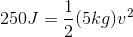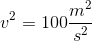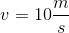# MCAT Physical : Other Rotational Motion Principles

## Example Questions

### Example Question #1 : Other Rotational Motion Principles

An eight inch long cylinder is composed of two substances: the first four inches are composed of aluminum, and the second four inches are composed of lead. The aluminum region has a mass of 30g and the lead region has a mass of 60g.

Where is the center of mass in the cylinder?

In the center of the cylinder, right in the middle of the length

In the center of the cylinder, one inch into the aluminum side

On the outside of the cylinder, right in the middle of the length

In the center of the cylinder, one inch into the lead side

In the center of the cylinder, one inch into the lead side

Explanation:

The center of mass is the point where we can consider all of the substance's mass to be concentrated. Finding the center of mass greatly facilitates mechanics problems, because we can perceive the force to be acting on only this one point.

Since the lead side of the cylinder is heavier than the aluminum side, we can conclude that the center of mass is located inside the lead side of the cylinder. Since the lead side has a mass of 60g, each inch of the lead side will have a mass of 15g. By going one inch into the lead side, there are 45g on each side of the point. As a result, we say that this is the location for the center of mass for the cylinder, as either side would weigh the same individually.

### Example Question #2 : Other Rotational Motion Principles

A body is in rotational equilibrium only if __________.

it is in free fall

its external torques sum to zero

It has zero angular momentum

its external forces sum to zero

its external torques sum to zero

Explanation:

A body achieves rotational equilibrium if it is rotating at a constant rate (or no rate, if it is at rest). Similarly, a body achieves translational equilibrium if it is moving at a constant rate (or no rate at all).

In order to impact translational equilibrium, an external force must be applied (Newton's first law). This force results in the acceleration of the mass (Newton's second law).

In order to impact rotational equilibrium, an external torque must be applied. This torque results in rotational acceleration by introducing a centripetal force.

An object can be in rotational equilibrium without translational equilibrium, and can be in translational equilibrium without rotational equilibrium. These two factors are independent in most cases.

### Example Question #3 : Other Rotational Motion Principles

A child of mass m stands at the outer edge of a carousel of radius R, which is rotating with angular velocity. If the child then moves inwards, to a distance of R/4 from the center of the carousel, which of the following statements regarding the child-carousel system is true?

The system’s angular momentum remains the same, and its angular velocity increases

The system’s angular momentum remains the same, and its moment of inertia increases

The system’s angular momentum decreases, and its moment of inertia remains constant

The system’s angular momentum decreases, and its moment of inertia also decreases

The system’s angular momentum remains the same, and its angular velocity decreases

The system’s angular momentum remains the same, and its angular velocity increases

Explanation:

Due to conservation of angular momentum, the angular momentum L must remain constant: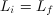.

Because of the child’s decreased distance from the axis of rotation, the moment of inertia of the system also decreases The child can be approximated as a point mass with moment of inertia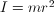, and the carousel’s moment of inertia remains unchanged. Moving the child toward the center of the carousel will decrease its moment of inertia.

To examine what happens to angular velocity, we need the definition of angular momentum,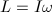. Replacing this in the conservation of angular momentum equation, we see that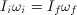. Since, then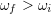so that the product remains the same.

Angular momentum remains the same, moment of inertia decreases, and angular velocity increases.

### Example Question #4 : Other Rotational Motion Principles

A carousel is rotating clockwise when observed from a bird's-eye view (looking directly down from above). What is the direction of the angular velocity vector,?

Upwards

Towards the axis of rotation

Downwards

Clockwise

Counterclockwise

Downwards

Explanation:

We can apply the right-hand rule for angular velocity: if the fingers on the right hand curl in the direction of rotation, the thumb points in the direction of the angular velocity vector. In this case the fingers curl clockwise, so thumb points downwards.

### Example Question #5 : Other Rotational Motion Principles

A 2kg mass is suspended on a rope that wraps around a frictionless pulley attached to the ceiling with a mass of 0.01kg and a radius of 0.25m. The other end of the rope is attached to a massless suspended platform, upon which 0.5kg weights may be placed. While the system is initially at equilibrium, the rope is later cut above the weight, and the platform subsequently raised by pulling on the rope.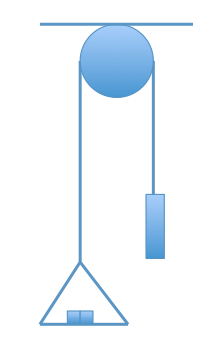If we assumed the pulley had internal friction, how would the angular acceleration of the pulley change?

Increase

Decrease

Remain the same

Not enough information to determine

Decrease

Explanation:

The angular acceleration would decrease. Newton’s second law states that F = ma. The net force, however, is reduced in the internal friction scenario because the friction acts to oppose the motion created by the rope around the pulley. As a side note, the pulley would also heat up because the energy change due to friction is released as heat.

### Example Question #6 : Other Rotational Motion Principles

Scientists studying the collision of particles note that a certain particle travels around a collider two times every second. If the tube's radius is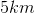, with what velocity is the particle traveling?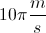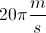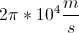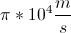Explanation:

The equation relating velocity and radius is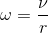.

Because angular velocity can also be found using the equation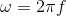, we can set the equations equal and solve for the velocity.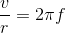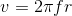Since we are given the radius as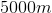and the frequency as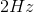, we can calculate the velocity. (Frequency can be found by the number of times an object travels around a full circle in one second).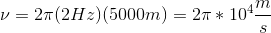Alternatively, we can use the radius to determine the circumference of the collider.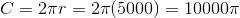The particle travels twice around the collider every second.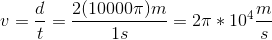### Example Question #7 : Other Rotational Motion Principles

A solid sphere, a hollow sphere, and a thin solid disk each have mass M and radius R and are released from rest at the top of a frictionless inclined plane. In which order do the objects reach the end of the inclined plane?

Assume that all objects roll, rather than slide.

1st: hollow sphere, 2nd: solid sphere, 3rd: disk

1st: disk, 2nd: hollow sphere, 3rd: solid sphere

1st: solid sphere, 2nd: disk, 3rd: hollow sphere

1st: disk, 2nd: solid sphere, 3rd: hollow sphere

1st: hollow sphere, 2nd: disk, 3rd: solid sphere

1st: solid sphere, 2nd: disk, 3rd: hollow sphere

Explanation:

An object with low moment of inertia will acquire less rotational kinetic energy and more translational kinetic energy (moves faster down the ramp) than an object with higher moment of inertia.

Moment of inertia depends on how close to the object's center of mass its mass is concentrated. An object with more of its mass close to the center will have lower moment of inertia, and subsequently more translational kinetic energy. The solid sphere has lowest moment of inertia, then the disk, and then the hollow sphere, thus the solid sphere reaches the end first, then the disk, and finally the hollow sphere.

### Example Question #8 : Other Rotational Motion Principles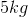ball rolls down a frictionless ramp with a height of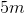. What is the velocity of the ball when it exits the ramp?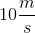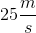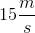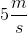Explanation:

Since the ramp is frictionless all the energy in the system is conserved. All the potential energy is converted into kinetic energy.

The potential energy is given by: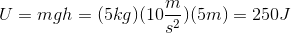The kinetic energy formula is: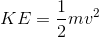Since all of the potential energy is converted to kinetic energy we can solve for velocity.